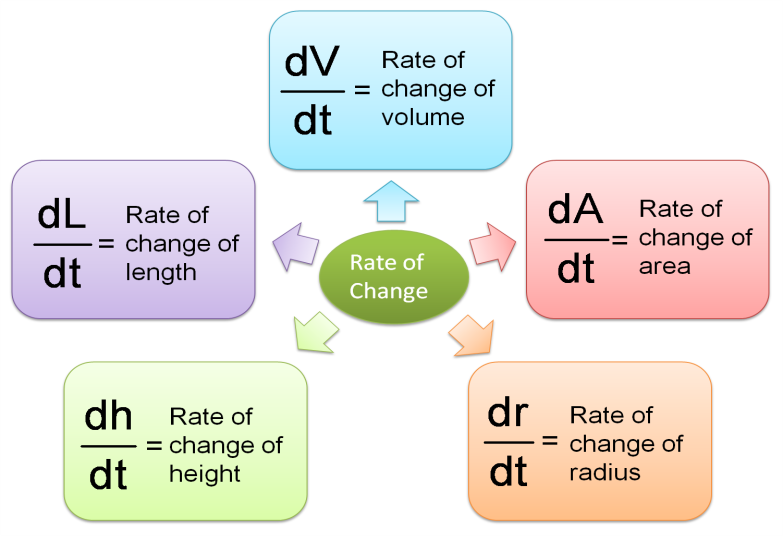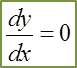# Short Questions (Question 20 – 22)

Question 20:
The volume of water V cm3, in a container is given by   $V=\frac{1}{5}{h}^{3}+7h$ , where h cm is the height of the water in the container. Water is poured into the container at the rate of 15cm3s-1. Find the rate of change of the height of water in cms-1, at the instant when its height is 3cm.

Solution:

Question 21:
A wire of length 88 cm is bent to form a circle. When the wire is heated, the length increases at the rate of 0.3 cms-1.
(a) Calculate the rate of change in the radius of the circle.
(b) Hence, calculate the radius of the circle after 5s.

Solution:

(a)

(b)

Question 22:

The diagram shows a conical container with diameter 0.8m and height 0.6m. Water is poured into the container at a constant rate of 0.02m3s-1. Calculate the rate of change of the height of the water level when the height of water level is 0.5m.

Solution:

# Short Questions (Question 14 – 16)

Question 14:
Given y = x (6 – x), express $y\frac{{d}^{2}y}{d{x}^{2}}+x\frac{dy}{dx}+18$ in terms of in the simplest form.

Hence, find the value of which satisfies the equation  $y\frac{{d}^{2}y}{d{x}^{2}}+x\frac{dy}{dx}+18=0$

Solution:

Question 15:
Find the coordinates of the point on the curve, y = (4x – 5)2 such that the gradient of the normal to the curve is $\frac{1}{8}$ .

Solution:
y = (4x – 5)2
$\frac{dy}{dx}$ = 2(4x – 5).4 = 32x – 40

Given the gradient of the normal is 1/8, therefore the gradient of the tangent is –8.
$\frac{dy}{dx}$ = –8
32x – 40 = –8
32x = 32
x = 1
y = (4(1) – 5)2= 1

Hence, the coordinates of the point on the curve, y = (4x – 5)2 is (1, 1).

Question 16:
A curve has a gradient function of kx2 – 7x, where k is a constant. The tangent to the curve at the point (1, 4) is parallel to the straight line y + 2x–1 = 0. Find the value of k.

Solution:
Gradient function of kx2– 7x is parallel to the straight line y + 2x–1 = 0
$\frac{dy}{dx}$ = kx2– 7x

y + 2x –1 = 0, y = –2x + 1, gradient of the straight line = –2
Therefore kx2– 7x = –2

At the point (1, 4),
k(1)2 – 7(1) = –2
k – 7 = –2
k = 5

# Short Questions (Question 11 – 13)

Question 11:
Given that the graph of function $f\left(x\right)=h{x}^{3}+\frac{k}{{x}^{2}}$  has a gradient function $f\text{'}\left(x\right)=12{x}^{2}-\frac{258}{{x}^{3}}$ such that h and are constants. Find the values of and k.

Solution:

Question 12:

Solution:

Question 13:

Solution:

# Short Questions (Question 5 – 7)

Question 5:
Given that f (x) = 3x2(4x 1)7, find f’(x).

Solution:
f (x) = 3x2(4x 1)7
f’(x) = 3x2. 7(4x 1)6. 8x + (4x 1)7. 6x
f’(x) = 168x3 (4x 1)6 + 6x (4x 1)7
f’(x) = 6x (4x 1)6 [28x2+ (4x 1)]
f’(x) = 6x (4x 1)6 (32x 1)

Question 6:
Given that y = (1 + 4x)3(3x 1)4, find $\frac{dy}{dx}$

Solution:
y = (1 + 4x)3(3x2 – 1)4
$\frac{dy}{dx}$
= (1 + 4x)3. 4(3x2 – 1)3.6x + (3x2 – 1)4. 3(1 + 4x)2.4
= 24x (1 + 4x)3(3x2 – 1)3 + 12 (3x2 – 1)4(1 + 4x)2
= 12 (1 + 4x)2(3x2 – 1)3 [2x (1 + 4x) + (3x2 – 1)]
= 12 (1 + 4x)2(3x2 – 1)3 [2x + 8x2 + 3x2 – 1]
= 12 (1 + 4x)2(3x2 – 1)3 [11x2 + 2x  – 1]

Question 7:
.

Solution:
$\begin{array}{l}f\left(x\right)=3x\sqrt{4{x}^{2}-1}=3x{\left(4{x}^{2}-1\right)}^{\frac{1}{2}}\\ f\text{'}\left(x\right)=3x.\frac{1}{2}{\left(4{x}^{2}-1\right)}^{-\frac{1}{2}}.8x+{\left(4{x}^{2}-1\right)}^{\frac{1}{2}}.3\\ f\text{'}\left(x\right)=12{x}^{2}{\left(4{x}^{2}-1\right)}^{-\frac{1}{2}}+3{\left(4{x}^{2}-1\right)}^{\frac{1}{2}}\\ f\text{'}\left(x\right)=3{\left(4{x}^{2}-1\right)}^{-\frac{1}{2}}\left[4{x}^{2}+\left(4{x}^{2}-1\right)\right]\\ f\text{'}\left(x\right)=\frac{3\left(8{x}^{2}-1\right)}{\sqrt{\left(4{x}^{2}-1\right)}}\end{array}$

# Short Questions (Question 1 – 4)

Question 1:
Differentiate the expression 2x (4x2 + 2x – 5) with respect to x.

Solution:
2x (4x2 + 2x – 5) = 8x3 + 4x2– 10x
$\frac{d}{dx}$ (8x3 + 4x2 – 10x)
= 24x + 8x –10

Question 2:
.

Solution:
$\begin{array}{l}y=\frac{{x}^{3}+2{x}^{2}+1}{3x}\\ y=\frac{{x}^{3}}{3x}+\frac{2{x}^{2}}{3x}+\frac{1}{3x}\\ y=\frac{{x}^{2}}{3}+\frac{2x}{3}+\frac{1}{3}{x}^{-1}\\ \frac{dy}{dx}=\frac{2x}{3}+\frac{2}{3}-\frac{1}{3}{x}^{-2}\\ \frac{dy}{dx}=\frac{2x}{3}+\frac{2}{3}-\frac{1}{3{x}^{2}}\end{array}$

Question 3:

Solution:
$\begin{array}{l}y=\frac{3}{\sqrt{5x+1}}=3{\left(5x+1\right)}^{-\frac{1}{2}}\\ \frac{dy}{dx}=-\frac{1}{2}.3{\left(5x+1\right)}^{-\frac{3}{2}}\left(5\right)\\ \frac{dy}{dx}=\frac{-15}{2{\left[{\left(5x+1\right)}^{3}\right]}^{\frac{1}{2}}}\\ \frac{dy}{dx}=\frac{-15}{2\sqrt{{\left(5x+1\right)}^{3}}}\end{array}$

Question 4:
Given that  $y=\frac{3}{5}{u}^{5}$ , where u = 4+ 1. Find $\frac{dy}{dx}$ in terms of x.

Solution:

# 9.9 Small Changes and Approximations

9.9 Small Changes and Approximations

,

This is very useful information in determining an approximation of the change in one variable given the small change in the second variable.

Example:
Given that y = 3x2 + 2x – 4. Use differentiation to find the small change in y when x increases from 2 to 2.02.

Solution:
$\begin{array}{l}y=3{x}^{2}+2x-4\\ \frac{dy}{dx}=6x+2\end{array}$

The small change in is denoted by δy while the small change in the second quantity that can be seen in the question is the x and is denoted by δx.

# Related Rates of Change

(A) Related Rates of Change

1. If two variables and y are connected by the equation y = f(x)

Notes:
If x changes at the rate of 5 cms -1   $\frac{dx}{dt}=5$
Decreases/leaks/reduces Þ  NEGATIVES values!!!

Example 1 (Rate of change of y and x)
Two variables, and y are related by the equation   $y=4x+\frac{3}{x}$ . Given that y increases at a constant rate of 2 units per second, find the rate of change of x when x = 3.

Solution:

(B) Rates of Change of Volume, Area, Radius, Height and Length(C) Rate of Change of Any Combination of Two Variables# 9.7 Second-Order Differentiation, Turning Points, Maximum and Minimum Points

(A) Second-Order Differentiation

1. When a function y = x3 + x2 – 3x + 6 is differentiated with respect to x, the derivative  $\frac{dy}{dx}=3{x}^{2}+2x-3$

2. The second function   $\frac{dy}{dx}$ can be differentiated again with respect to x. This is called the second derivative of y with respect to and can be written as $\frac{{d}^{2}y}{d{x}^{2}}$ .

3. Take note that   $\frac{{d}^{2}y}{d{x}^{2}}\ne {\left(\frac{dy}{dx}\right)}^{2}$ .

For example,
If y = 4x3 – 7x2 + 5x – 1,

The first derivative   $\frac{dy}{dx}=12{x}^{2}-14x+5$

The second derivative    $\frac{{d}^{2}y}{d{x}^{2}}=24x-14$

(B) Turning Points, Maximum and Minimum Points(a) At Turning Points A and B,(b) At Maximum Point A(c) At Minimum Point B,# 9.6 Gradients of Tangents, Equations of Tangent and Normal

9.6 Gradients of Tangents, Equations of Tangents and NormalsIf A(x1, y1) is a point on a line y = f(x), the gradient of the line (for a straight line) or the gradient of the tangent of the line (for a curve) is the value of $\frac{dy}{dx}$ when x = x1.

(A) Gradient of tangent at A(x1, y1):(B) Equation of tangent:(C) Gradient of normal at A(x1, y1):(D) Equation of normal :Example 1 (Find the Equation of Tangent)
Given that $y=\frac{4}{{\left(3x-1\right)}^{2}}$ . Find the equation of the tangent at the point (1, 1).

Solution:

Example 2 (Find the Equation of Normal)
Find the gradient of the curve $y=\frac{7}{3x+4}$ at the point (-1, 7). Hence, find the equation of the normal to the curve at this point.

Solution:

# 9.5 First Derivatives of Composite Function

(A) Differentiate Composite Function using Chain Rule

Example:
Differentiate y = (x2– 1)8 .

Solution:

(B) Differentiate Composite Function using Alternative Method
- Easy Version

Example:
Differentiate y = (x2 – 1)8 .

Solution:
$\begin{array}{l}y={\left({x}^{2}-1\right)}^{8}\\ \frac{dy}{dx}=8{\left({x}^{2}-1\right)}^{7}\frac{d}{dx}\left({x}^{2}-1\right)\\ \frac{dy}{dx}=8{\left({x}^{2}-1\right)}^{7}\left(2x\right)\\ \frac{dy}{dx}=16x{\left({x}^{2}-1\right)}^{7}\end{array}$

Practice 1:

Solution:
$\begin{array}{l}y=\frac{1}{3x-7}={\left(3x-7\right)}^{-1}\\ \frac{dy}{dx}=-1{\left(3x-7\right)}^{-2}.3\\ \frac{dy}{dx}=\frac{-3}{{\left(3x-7\right)}^{2}}\end{array}$

Practice 2:

Solution:
$\begin{array}{l}y=\sqrt{2{x}^{2}-5x+1}={\left(2{x}^{2}-5x+1\right)}^{\frac{1}{2}}\\ \frac{dy}{dx}=\frac{1}{2}{\left(2{x}^{2}-5x+1\right)}^{-\frac{1}{2}}\left(4x-5\right)\\ \frac{dy}{dx}=\frac{4x-5}{2\sqrt{2{x}^{2}-5x+1}}\end{array}$